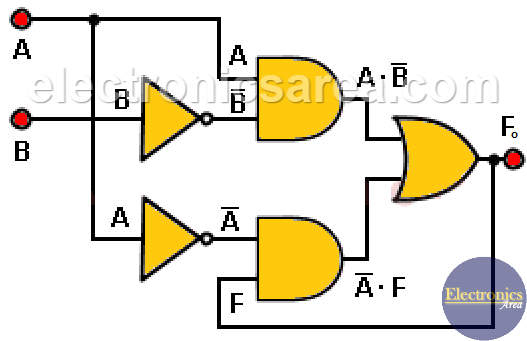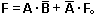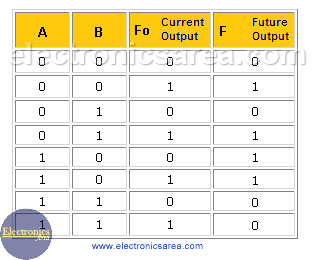# What is a sequential circuit?

This tutorial explains how a sequential circuit made with gates works. There are other circuits or ICs that already work sequential way, like a flip-flop. For example, JK flip-flop, T flip-flop, D flip-flop, SR flip-flop, etc.

The main difference between combinational circuits and sequential circuits is that in sequential circuits there is one or more output signals fed back to one or more inputs to obtain a new output.

## Sequential circuit example using gates

Looking at the following diagram, you can see that the output of the OR gate is feed backed to the input of the lower AND gate. This means that the future F output (F) depends on the inputs A and B and on the value of the current F output. (Fo)

In other words, the F output (future output)  depends on the A and B inputs, and the value that the Fo output.## Sequential circuit Truth table

Truth table would generally be like the one below, where the inputs are: A, B and the current output (Fo). The output F is the future output. (look at the following truth table)It can be seen from the previous truth table that the last column “future F output” is sometimes different from the “current F output”, because the “future output F” not only depends on the current A and B inputs but also that also from the “current output Fo”.

This diagram and its respective truth table are a specific example. Other diagrams can have more inputs A, B, C, … etc), more outputs (F1, F2, F3, …, etc) and more feedback outputs (F1, F2, F3, …, etc).

In case of having more outputs, it would be necessary to obtain a truth table for each “future F output” according to the existing inputs and all the “current F outputs” that are fed back.

Notes:

• It is important to know that although the current F output column (Fo) shows “0” and “1” for all combinations of A and B, this is not necessarily true.
• The outputs in other sequential circuits can be anywhere within the circuit diagram.
• 2
•
• 3
•
•
•
•
•
5
Shares
•
5
Shares
• 2
•
• 3
•
•
•
•